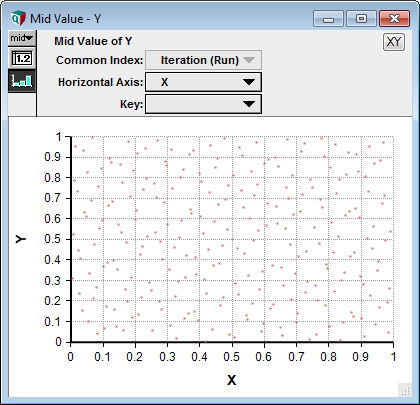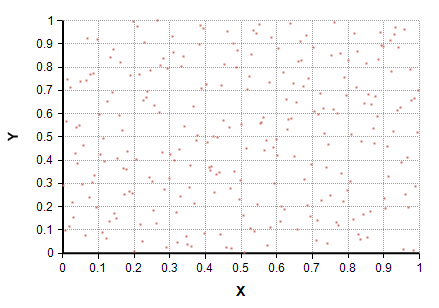# SobolSequence

## SobolSequence(n, i)

A Sobol sequence is a sequence of points intended to cover a multi-dimensional space more evenly than a purely random Monte Carlo sequence. This function returns the «n»th Sobol sequence along index «i», where «i» defaults to the Run index. «n» is a scalar (positive integer) and the result is indexed by «i».

## Example

SampleSize := 256
Variable X := SobolSequence(1)
Variable Y := SobolSequence(2)

Here is a scatter plot of Y vs. X:The Sobol sequence tends to fill the 2-D space more evenly than a purely Uniform random scatter:The Sobol has fewer instances of several points clustering closely or of large regions containing no points.

## Description

Given a set of j positive integers, n1, n2, ... nj, the sequences SobolSequence(n1), SobolSequence(n2), ..., SobolSequence(nj) tend to fill the j-dimensional unit hypercube in a manner that provides uniform coverage.

The sequences returned as «n» increases is defined by Analytica, but use successively higher-order Sobol polynomials as «n» increases. Internal polynomials are defined up from «n» equals 1 to 21,000.

## SobolSequence(n, nEncodesPolynomial: True)

You can specify the optional parameter «nEncodesPolynomial» as true to supply your own primitive polynomial. The bits of «n» then encode the coefficients of the primitive polynomial, with the highest order bit encoding the highest order polynomial term, and the constant 1 not included in the encoding. For example, the polynomial

$\displaystyle{ x^4 + x^3 + 1 }$

is encoded as 0b1100.